Publicité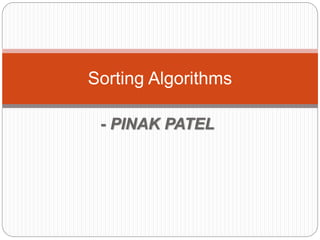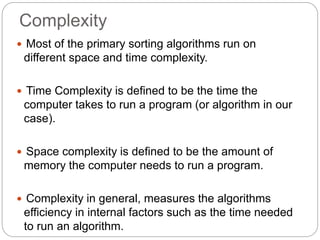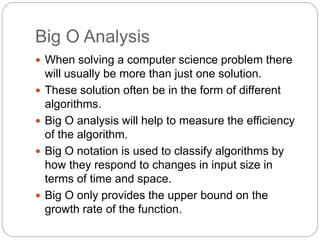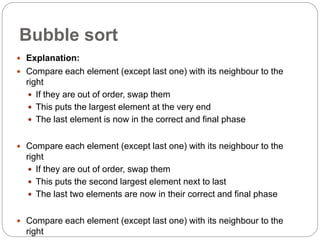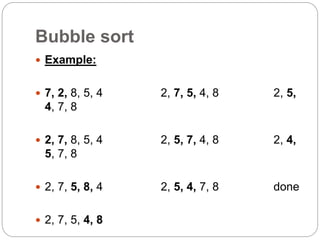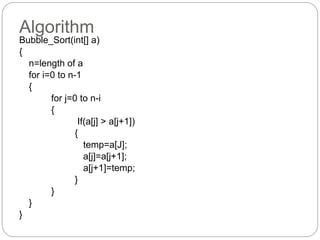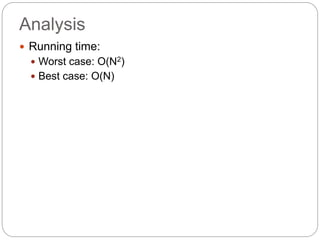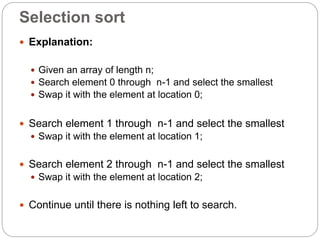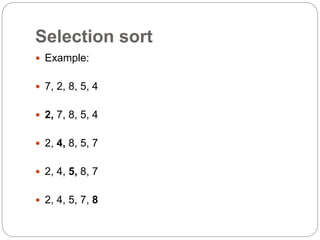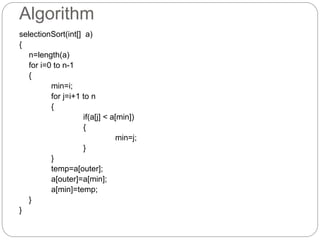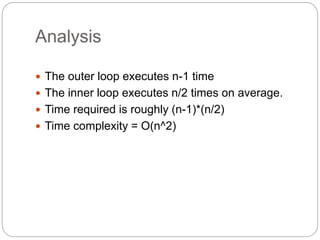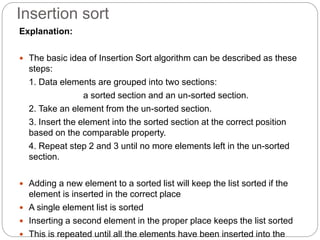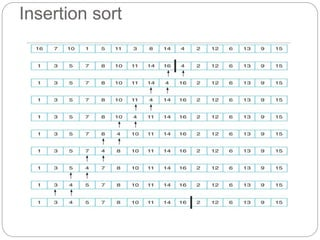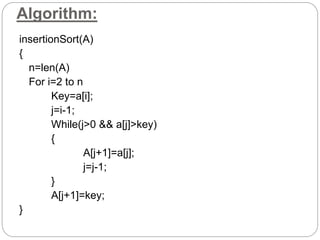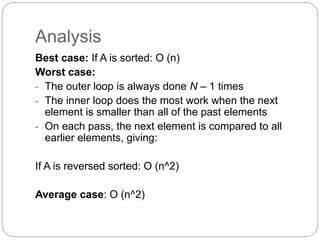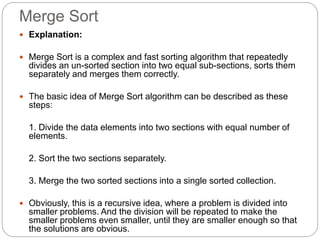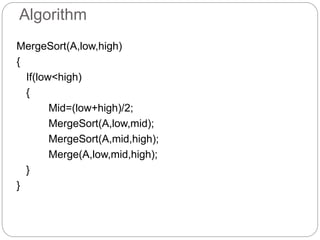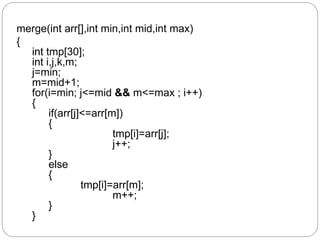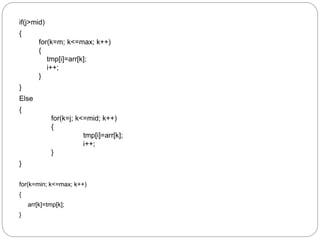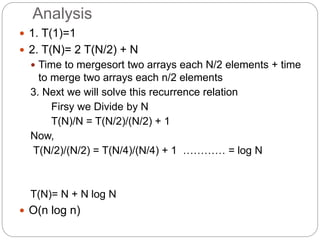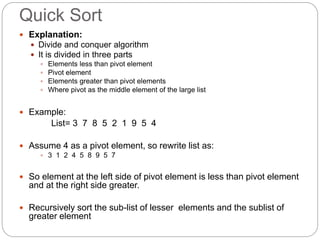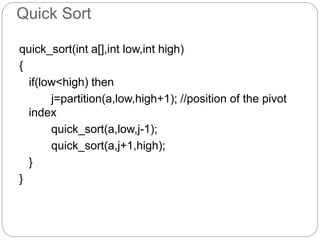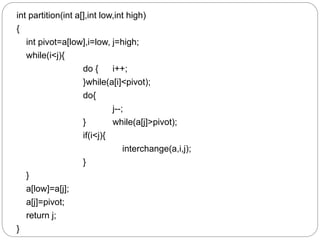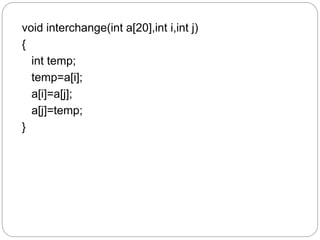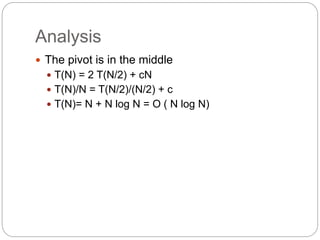1 sur 26
Publicité

### Sorting pnk

1. - PINAK PATEL Sorting Algorithms
2. Complexity  Most of the primary sorting algorithms run on different space and time complexity.  Time Complexity is defined to be the time the computer takes to run a program (or algorithm in our case).  Space complexity is defined to be the amount of memory the computer needs to run a program.  Complexity in general, measures the algorithms efficiency in internal factors such as the time needed to run an algorithm.
3. Big O Analysis  When solving a computer science problem there will usually be more than just one solution.  These solution often be in the form of different algorithms.  Big O analysis will help to measure the efficiency of the algorithm.  Big O notation is used to classify algorithms by how they respond to changes in input size in terms of time and space.  Big O only provides the upper bound on the growth rate of the function.
4. Bubble sort  Explanation:  Compare each element (except last one) with its neighbour to the right  If they are out of order, swap them  This puts the largest element at the very end  The last element is now in the correct and final phase  Compare each element (except last one) with its neighbour to the right  If they are out of order, swap them  This puts the second largest element next to last  The last two elements are now in their correct and final phase  Compare each element (except last one) with its neighbour to the right
5. Bubble sort  Example:  7, 2, 8, 5, 4 2, 7, 5, 4, 8 2, 5, 4, 7, 8  2, 7, 8, 5, 4 2, 5, 7, 4, 8 2, 4, 5, 7, 8  2, 7, 5, 8, 4 2, 5, 4, 7, 8 done  2, 7, 5, 4, 8
6. Algorithm Bubble_Sort(int[] a) { n=length of a for i=0 to n-1 { for j=0 to n-i { If(a[j] > a[j+1]) { temp=a[J]; a[j]=a[j+1]; a[j+1]=temp; } } } }
7. Analysis  Running time:  Worst case: O(N2)  Best case: O(N)
8. Selection sort  Explanation:  Given an array of length n;  Search element 0 through n-1 and select the smallest  Swap it with the element at location 0;  Search element 1 through n-1 and select the smallest  Swap it with the element at location 1;  Search element 2 through n-1 and select the smallest  Swap it with the element at location 2;  Continue until there is nothing left to search.
9. Selection sort  Example:  7, 2, 8, 5, 4  2, 7, 8, 5, 4  2, 4, 8, 5, 7  2, 4, 5, 8, 7  2, 4, 5, 7, 8
10. Algorithm selectionSort(int[] a) { n=length(a) for i=0 to n-1 { min=i; for j=i+1 to n { if(a[j] < a[min]) { min=j; } } temp=a[outer]; a[outer]=a[min]; a[min]=temp; } }
11. Analysis  The outer loop executes n-1 time  The inner loop executes n/2 times on average.  Time required is roughly (n-1)*(n/2)  Time complexity = O(n^2)
12. Insertion sort Explanation:  The basic idea of Insertion Sort algorithm can be described as these steps: 1. Data elements are grouped into two sections: a sorted section and an un-sorted section. 2. Take an element from the un-sorted section. 3. Insert the element into the sorted section at the correct position based on the comparable property. 4. Repeat step 2 and 3 until no more elements left in the un-sorted section.  Adding a new element to a sorted list will keep the list sorted if the element is inserted in the correct place  A single element list is sorted  Inserting a second element in the proper place keeps the list sorted  This is repeated until all the elements have been inserted into the
13. Insertion sort
14. Algorithm: insertionSort(A) { n=len(A) For i=2 to n Key=a[i]; j=i-1; While(j>0 && a[j]>key) { A[j+1]=a[j]; j=j-1; } A[j+1]=key; }
15. Analysis Best case: If A is sorted: O (n) Worst case: - The outer loop is always done N – 1 times - The inner loop does the most work when the next element is smaller than all of the past elements - On each pass, the next element is compared to all earlier elements, giving: If A is reversed sorted: O (n^2) Average case: O (n^2)
16. Merge Sort  Explanation:  Merge Sort is a complex and fast sorting algorithm that repeatedly divides an un-sorted section into two equal sub-sections, sorts them separately and merges them correctly.  The basic idea of Merge Sort algorithm can be described as these steps: 1. Divide the data elements into two sections with equal number of elements. 2. Sort the two sections separately. 3. Merge the two sorted sections into a single sorted collection.  Obviously, this is a recursive idea, where a problem is divided into smaller problems. And the division will be repeated to make the smaller problems even smaller, until they are smaller enough so that the solutions are obvious.
17. Algorithm MergeSort(A,low,high) { If(low<high) { Mid=(low+high)/2; MergeSort(A,low,mid); MergeSort(A,mid,high); Merge(A,low,mid,high); } }
18. merge(int arr[],int min,int mid,int max) { int tmp; int i,j,k,m; j=min; m=mid+1; for(i=min; j<=mid && m<=max ; i++) { if(arr[j]<=arr[m]) { tmp[i]=arr[j]; j++; } else { tmp[i]=arr[m]; m++; } }
19. if(j>mid) { for(k=m; k<=max; k++) { tmp[i]=arr[k]; i++; } } Else { for(k=j; k<=mid; k++) { tmp[i]=arr[k]; i++; } } for(k=min; k<=max; k++) { arr[k]=tmp[k]; }
20. Analysis  1. T(1)=1  2. T(N)= 2 T(N/2) + N  Time to mergesort two arrays each N/2 elements + time to merge two arrays each n/2 elements 3. Next we will solve this recurrence relation Firsy we Divide by N T(N)/N = T(N/2)/(N/2) + 1 Now, T(N/2)/(N/2) = T(N/4)/(N/4) + 1 ………… = log N T(N)= N + N log N  O(n log n)
21. Quick Sort  Explanation:  Divide and conquer algorithm  It is divided in three parts  Elements less than pivot element  Pivot element  Elements greater than pivot elements  Where pivot as the middle element of the large list  Example: List= 3 7 8 5 2 1 9 5 4  Assume 4 as a pivot element, so rewrite list as:  3 1 2 4 5 8 9 5 7  So element at the left side of pivot element is less than pivot element and at the right side greater.  Recursively sort the sub-list of lesser elements and the sublist of greater element
22. Quick Sort quick_sort(int a[],int low,int high) { if(low<high) then j=partition(a,low,high+1); //position of the pivot index quick_sort(a,low,j-1); quick_sort(a,j+1,high); } }
23. int partition(int a[],int low,int high) { int pivot=a[low],i=low, j=high; while(i<j){ do { i++; }while(a[i]<pivot); do{ j--; } while(a[j]>pivot); if(i<j){ interchange(a,i,j); } } a[low]=a[j]; a[j]=pivot; return j; }
24. void interchange(int a,int i,int j) { int temp; temp=a[i]; a[i]=a[j]; a[j]=temp; }
25. Analysis  The pivot is in the middle  T(N) = 2 T(N/2) + cN  T(N)/N = T(N/2)/(N/2) + c  T(N)= N + N log N = O ( N log N)
26. THANK YOU
Publicité